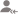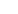true

Take Class 12 Tuition from the Best Tutors

•Affordable fees
•1-1 or Group class
•Flexible Timings
•Verified Tutors

Search in# Learn Mathematics with Free Lessons & Tips

Post a Lesson

All

All

Lessons

DiscussionProper Understanding and Constant Practice will make you Pro

2*4=2+3(4)2=26 Substitute values of a and b in the given function and compute its value
Dislike Bookmark

Every diagonal element of a skew symmetric matrix is

If the matrix A is both symmetric and skews symmetric, then A will be.

Take Class 12 Tuition from the Best Tutors

•Affordable fees
•Flexible Timings
•Choose between 1-1 and Group class
•Verified Tutors

Matrices A and B will be inverse of each other only if

A Relation R:AàA is said to be Symmetric if ———- a,b,A

Define universal relation? Give example.

Take Class 12 Tuition from the Best Tutors

•Affordable fees
•Flexible Timings
•Choose between 1-1 and Group class
•Verified Tutors

Prove that the function f: R à R, given by f(x) = 2x, is one – one.

Find the value of cos-1 (1/2) + 2 sin-1 (1/2).

If tan-1 (x − 1)/(x − 2) + tan-1 (x + 1)/(x + 2) = 𝜋/4, then find the value of x.

Take Class 12 Tuition from the Best Tutors

•Affordable fees
•Flexible Timings
•Choose between 1-1 and Group class
•Verified Tutors

Find the principal value of tan-1 (1).

UrbanPro.com helps you to connect with the best Class 12 Tuition in India. Post Your Requirement today and get connected.

Overview

Questions 615

Lessons 54

Total Shares126,578 Followers

## Top Contributors

Connect with Expert Tutors & Institutes for Mathematics

## Class 12 Tuition in:

x

X

### Looking for Class 12 Tuition Classes?

The best tutors for Class 12 Tuition Classes are on UrbanPro

• Select the best Tutor
• Book & Attend a Free Demo
• Pay and start Learning### Take Class 12 Tuition with the Best Tutors

The best Tutors for Class 12 Tuition Classes are on UrbanPro